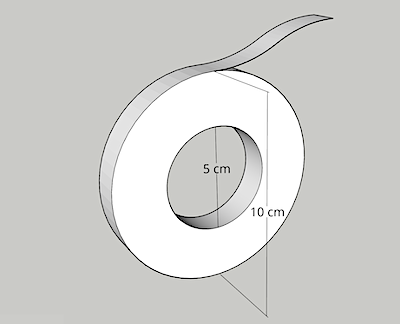# Neil's News

### ChatGPT vs Math

17 February 2023

There is a roll of tape. The tape is 100 meters long when unrolled. When rolled up, the outer diameter is 10 cm, and the inner diameter is 5 cm. How thick is the tape?If this sounds like fun, stop reading here and work it out yourself. Otherwise, here's my sample solution, followed by ChatGPT's "solution" and some of Google Bard's opinions.

First let's calculate the side surface area of the tape on the roll. That's the area of a circle 5 cm in radius, minus a circle 2.5 cm in radius. The formula for the area of a circle is A = π r². So the tape's area is π 5 cm² - π 2.5 cm² = 58.9 cm².

Second, we must recognise that the side surface area of the tape doesn't change when it is unrolled, it simply becomes a rectangle 10,000 cm (100 meters) long. Dividing 58.9 cm² (the area) by 10,000 cm (the length) results in a tape thickness of 0.00589 cm.

### ChatGPT

At the time of writing, ChatGPT is in the news. So I asked it to solve the tape problem. Here's its response, with my annotations.

To find the thickness of the tape, we can use the formula for the volume of a cylinder, which is:

V = π * r^2 * h

where V is the volume, r is the radius, and h is the height.

The radius of the inner cylinder is given as 5 cm / 2 = 2.5 cm = 0.025 m

The radius of the outer cylinder is given as 10 cm / 2 = 5 cm = 0.05 m

Good job on converting everything to the same units.

The height of the cylinder is given as 100 m.

There's the first mistake: a comprehension issue. The height of the cylinder was not provided, nor is it relevant. So we'll note that error and keep going.

So the volume of the outer cylinder is:

V_outer = π * 0.05^2 * 100 = 78.5398 m^3

The volume of the inner cylinder is: V_inner = π * 0.025^2 * 100 = 19.6375 m^3

Now we can find the volume of the tape by subtracting the volume of the inner cylinder from the volume of the outer cylinder:

V_tape = V_outer - V_inner = 78.5398 - 19.6375 = 58.9023 m^3

All the math here is correct.

To find the thickness of the tape, we will divide the volume of the tape by the surface area of the tape.

The surface area of the tape is the difference between the surface area of the outer cylinder and the surface area of the inner cylinder

So the surface area of the tape is 2πr*h where r is the difference between outer radius and inner radius and h is the height.

I can't map any of this to real-world understanding.

r = 0.05-0.025 = 0.025 h = 100m

Surface area of the tape = 2π0.025*100 = 0.7854 m^2

This math is not only non-sensical, it is also wrong. 2π0.025*100 = 15.7.

Now we can divide the volume of the tape by the surface area of the tape to find the thickness of the tape

Thickness of the tape = V_tape / Surface area of the tape = 58.9023 m^3 / 0.7854 m^2 = 74.9 m

So, the thickness of the tape is 74.9 meters.

Only out by seven orders of magnitude. But said with confidence!